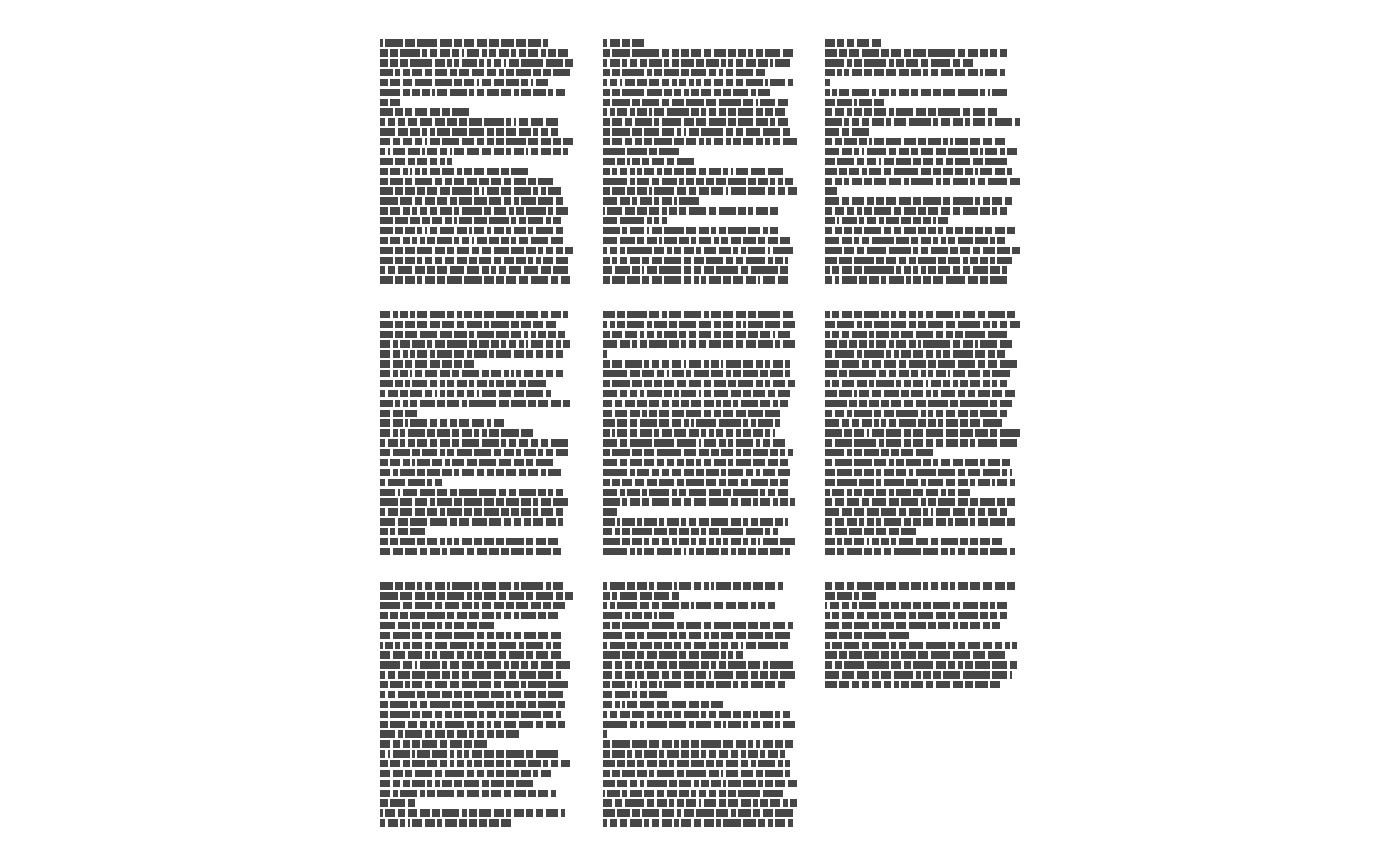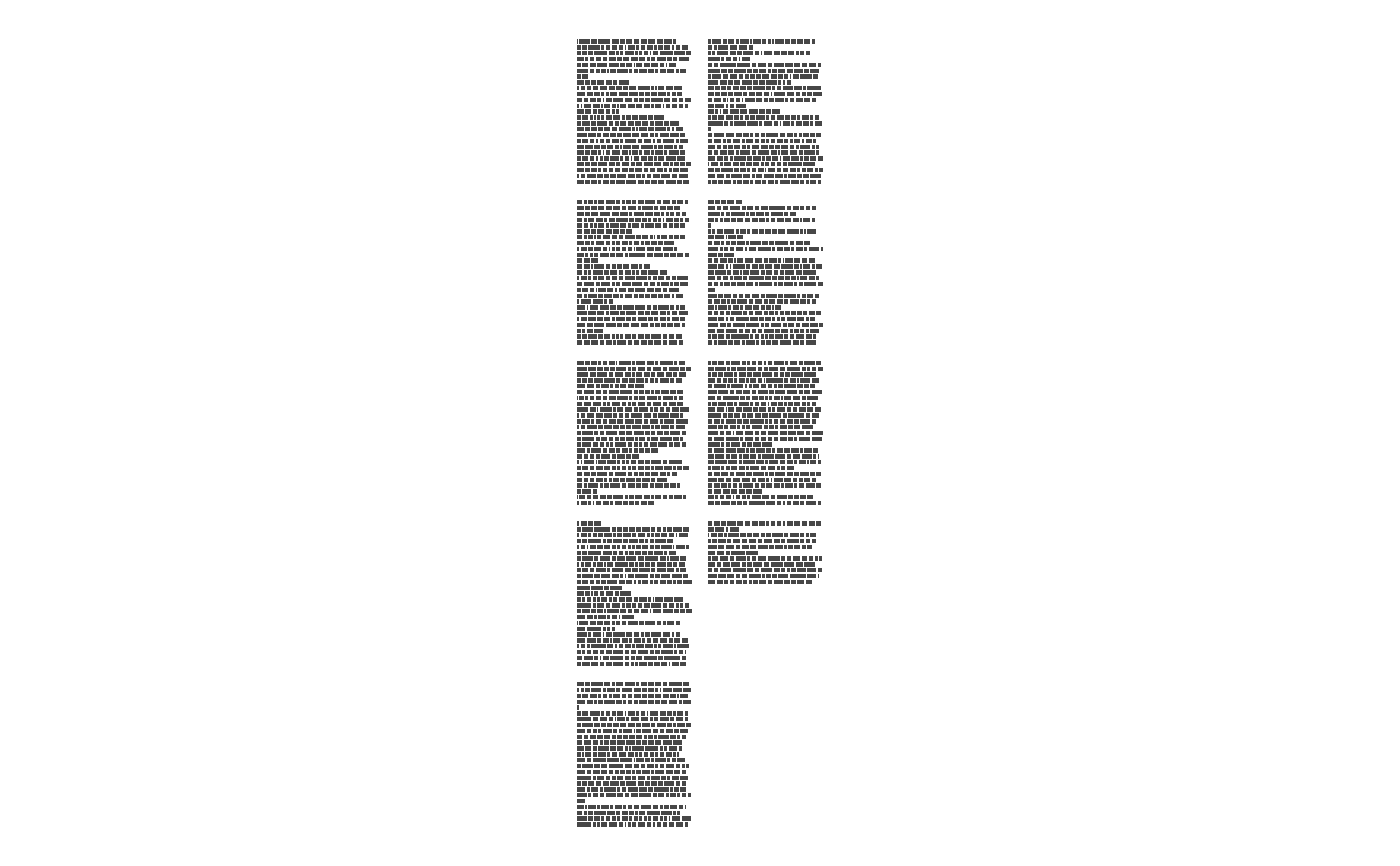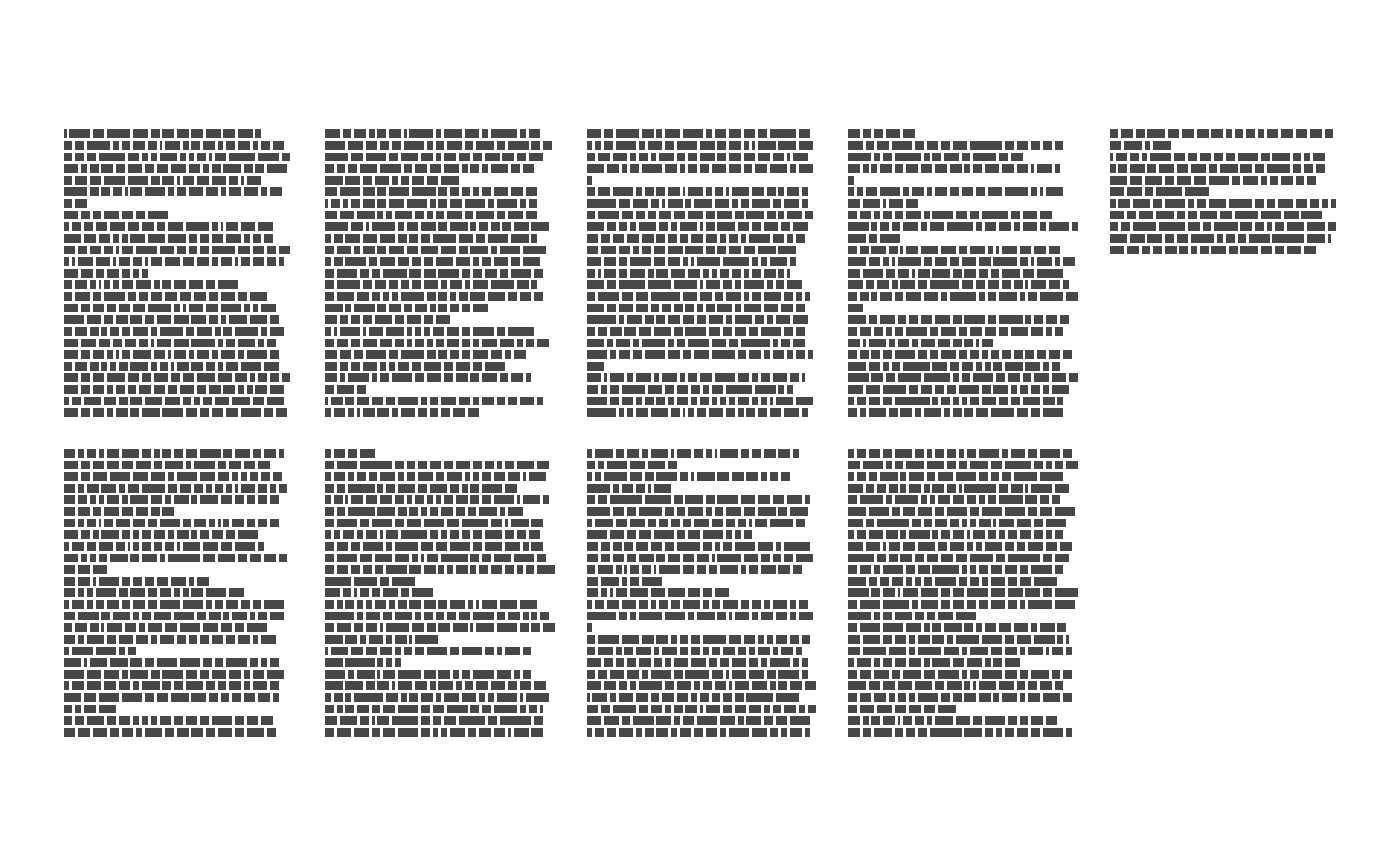Creates a quick visualization of the page layout

ggpage_quick(book, lpp = 25, character_height = 3,
vertical_space = 1, x_space_pages = 10, y_space_pages = 10,
nrow = NULL, ncol = NULL, bycol = TRUE)

## Arguments

book Character or data.frame. Can either have each element be a separate line or having each element being separate words. Numeric. Lines Per Page. Number of lines allocated for each page. Numeric. Relative size of the height of each letter compared to its width. Numeric. Distance between each lines vertically. Numeric. Distance between pages along the x-axis. Numeric. Distance between pages along the y-axis. Numeric. Number of rows of pages, if omitted defaults to square layout. Numeric. Number of columns of pages, if omitted defaults to square layout. Logical. If TRUE (the default) the matrix is filled by columns, otherwise the matrix is filled by rows.

## Value

A ggplot object with the given visualization.

## Examples

library(dplyr)
library(stringr)
library(ggplot2)
library(tidytext)
library(ggpage)
# quick
## data.frame with full lines
ggpage_quick(tinderbox)## vector with full lines
ggpage_quick(tinderbox %>%
pull(text))#> Error in check_input(x): Input must be a character vector of any length or a list of character
#>   vectors, each of which has a length of 1.## data.frame with single words
ggpage_quick(tinderbox %>%
unnest_tokens(text, text))#> Error in check_input(x): Input must be a character vector of any length or a list of character
#>   vectors, each of which has a length of 1.## vector with single words
ggpage_quick(tinderbox %>%
unnest_tokens(text, text) %>%
pull(text))#> Error in check_input(x): Input must be a character vector of any length or a list of character
#>   vectors, each of which has a length of 1.
# nrow and ncol
ggpage_quick(tinderbox, nrow = 2)ggpage_quick(tinderbox, ncol = 2)